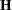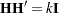A Hadamard matrixis a square matrix whose elements are either 1 or –1 such thatwhereis the dimension ofandis the identity matrix of order. The orderis necessarily 1, 2, or a positive integer that is a multiple of 4.
For example, the following matrix is a Hadamard matrix of dimension= 8: